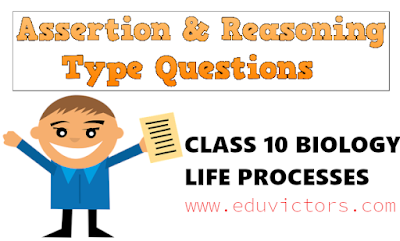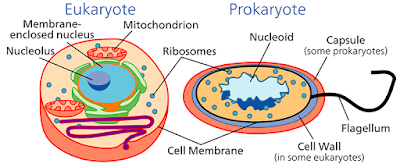## Class 7 - Mathematics - Properties of Integers

Integers are closed under addition, i.e. for any two integers,a and b, a+b is an integer.
eg: 3 + 4 = 7
-9 + 7 = 2

2. Closure Property under Subtraction:

Integers are closed under subtraction, i.e. for any two integers,a and b, a-b is an integer.
eg:
-21 -(-9) = -12
8  -3    = 5

3. Closure Property under Multiplication:

Integers are closed under multiplication, i.e. for any two integers,a and b, ab is an integer.
e.g.
5 × 6 = 30
-3 × -8 = 24
-3 × 7 = -21## Wednesday, 1 May 2019

### CBSE Class 10 - Maths - Chapter Polynomials - 1 Mark Questions (#cbsenotes)(#class10maths)(#eduvictors)

Chapter Polynomials - 1 Mark Questions
Class 10 - Maths

Q1: If α and β are the roots of ax² -bx + c (a ≠ 0), compute α + β?

Q2:  If one zero of the quadratic polynomial x² + 3x + k is 2, then the value of k is?

Q3:  Given that two of the zeroes of the cubic polynomial ax³ + bx² + cx + d are 0, find the third zero?

Q4: In the given figure for a given polynomial p(x), find the number of zeroes.## Amazing Human Body - Quick Facts

1.  The hardest bone in the human body is the jawbone.

2.  The number of eye blinks varies greatly from about 29 blinks each minute if you are talking to someone to only 4 blinks each minute if you are reading.

3.  The average human blinks 25 times per minute.

4.  A nail takes around 6 months to grow from base to the tip.

5.  Each second 10,000,000 cells die and are replaced in your body.## Integers and Operations On Integers

Class 7 - Mathematics

1. When two positive integers are added, we get a positive integer.
e.g. 6 + 4 = 10

2. When two negative integers are added, we get a negative integer.
e.g.  -6 + (-3) = -9

3. When a positive and a negative integer are added, the sign of the sum is always the sign of the bigger number of the two, without considering their signs.
e.g.  45 + -25 = 20   and    -45 + 20 = -25

The additive inverse of any integer a is - a, and the additive inverse of (- a) is a.
e.g.  Additive inverse of (-15) = - (-15) = 15## Chemical Reactions and Equations

MCQs & Other Resources
Class 10 - Chemistry

The transformation of chemical substance into a new chemical substance by making and breaking of bonds between different atoms is known as Chemical Reaction.  Chemical equations are balanced to satisfy the law of conservation of mass.

Here are MCQs, study notes and other useful links:## Class 10 - Biology Chapter: Life Processes Assertion Reasoning Type Questions

From session 2019-20 onwards, CBSE introduces new pattern of questions which are assertion and reasoning based.

The questions below consist of statements of an Assertion and a Reason. Use the following key to choose appropriate answer:

(A) If both assertion and reason are CORRECT and reason is the CORRECT explanation of the assertion.

(B) If both assertion and reason are CORRECT, but reason is NOT THE CORRECT explanation of the assertion.

(C) If assertion is CORRECT, but reason is INCORRECT

(D) If assertion is INCORRECT, but reason is CORRECT

(E) If both assertion and reason are INCORRECT

QUESTIONS:

1. ASSERTION: Molecular movements are needed for life.
REASON: Body structures made up of these molecules need continuous repair and maintenance.## Cell Membrane - Structure and Functions

Class 9 - Biology - Cell: Fundamental Unit Of LifePlasma Membrane DiagramImage Credits: Ruth Lawson. Otago Polytechnic Wikimedia

Structure:
1. Cell membrane is also called as plasma membrane or plasma lemma.

2. It is the limiting boundary of each cell which separates the cytoplasm from its surroundings.
3. It is found in both plant as well as animal cells.
4. It is the outermost covering of a cell in case of animals and lies below the cell wall in case of plants.## Tuesday, 23 April 2019

### Class 8 - Science - Chapter - Coal and Petroleum - Very Short Questions and Answers (#cbsenotes)(#eduvictors)

Class 8 - Science

## Coal and Petroleum

Q1: Name two each of the exhaustible and inexhaustible natural resources.

Ans: Petroleum and Coal

Q2: Write the name of two natural resources other than coal and petroleum.

Ans: Air, Forests, and Water

Q3: Why are air, water, and soil called natural resources?

Ans: Air, water, and soil are provided by nature, so they are called natural resources.

Q4: Why are coal, petroleum and natural gas called fossil fuels?

Ans: Coal, petroleum and natural gas are formed by fossils, so they are called fossil fuels.

Q5: What are fossil fuels?

Ans: Fossil fuels are the energy-rich components of carbon which were made by the decomposition of plants and animals buried under the earth, long-long ago.## Cell: Fundamental Unit Of Life -

Very Short Answers Based Questions (Set-1)
Class 9 BiologyCell Types: Prokaryotes and Eukaryotes Image credits: Wikimedia

1. Name the largest cell of living world ?

2. Amoeba is a _______________ organism.

3. Who gave the fluid mosaic model of plasma membrane?

4. Movement of solvent into the cell is called as_____________ .

5. Which cell organelle is called as the ‘Head quarter of cell’?## Class 10 - Biology - Life Processes -  Nutrition in Human BeingsHuman Alimentary Canal Image Credits: clker.com

Q1: What is common name of buccal cavity?

Answer: _ _ _ _ _ _ _ _ _ _ _ _ _ _ _ _ _ _ _ _ _ _ _ _ _ _ _ _ _ _ _ _ _ _ _ _ _ _ _ _ _ _ _ _ _

Q2: Name the enzyme present in saliva?

Answer: _ _ _ _ _ _ _ _ _ _ _ _ _ _ _ _ _ _ _ _ _ _ _ _ _ _ _ _ _ _ _ _ _ _ _ _ _ _ _ _ _ _ _ _ _

Q3: Which organ releases gastric juice?

Answer: _ _ _ _ _ _ _ _ _ _ _ _ _ _ _ _ _ _ _ _ _ _ _ _ _ _ _ _ _ _ _ _ _ _ _ _ _ _ _ _ _ _ _ _ _

Q4: Name the digestive juice(s) received by small intestine.

Answer: _ _ _ _ _ _ _ _ _ _ _ _ _ _ _ _ _ _ _ _ _ _ _ _ _ _ _ _ _ _ _ _ _ _ _ _ _ _ _ _ _ _ _ _ _

Q5: Name the largest digestive gland.

Answer: _ _ _ _ _ _ _ _ _ _ _ _ _ _ _ _ _ _ _ _ _ _ _ _ _ _ _ _ _ _ _ _ _ _ _ _ _ _ _ _ _ _ _ _ _